Next: Method schwabisch Up: Implementation Previous: Method ambiguity   Contents

## Method rodriguez

Use same definitions as exact method, see also Annex D. See also . This method uses the geometry to compute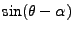. There are two errors in it for now. First H is not computed exact. Second the baseline parameters are not computed exact per line. P is evaluated at reference surface and S according to that position. This means that if the orbits are not parallel the point S is not computed correctly, which introduces errors in the baseline computation. The co-registration model is better used for that.

Known:
r1 range to M,P
position M
B baseline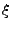baseline orientation with regard to (equals our def. of alpha)

Compute:
theta (angle state, look: with formulas exact (no iterations)
H height sat above some surface ?? how to compute this exact??

The following we derived for our baseline definition: (beta = angle (2-1,P-1) counterclockwise:)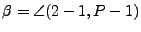(11)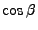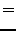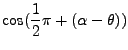(12)(13)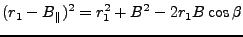(14)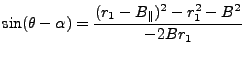(15)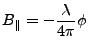(16)

So theta can be solved for exact with these formulas. Note: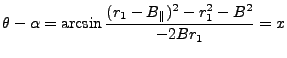(17)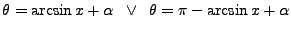(18)

I did not find an efficient way to always use the right expression yet. Now I use the fact that theta is about 20 degrees, but it should be possible to find out quadrants.

Compute H from known: theta, position Master (rho1), r1 In triangle (1,P,0) three Start with cosine law for line across theta = p(19)

Then compute cosine of angle mu across r1 in same triangle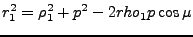(20)

Unclear how to compute H exact. for now use approximation. (set radius of earth at location of satellite equal to radius at location of P) compute satellite height by Bowring's method (xyz2ell) then radius R of earth at phi,lambda to satellite: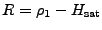(21)

Approximate H in this way(22)

Compute error of this approximation (preliminary study!):

This will cause a bias and some trend in the height. Because there likely already is a trend due to orbit errors, this is not as bad as it might seem. By using tie points a good height may be computed. For now we did not implement a routine that uses tie points.

New way of computing H: (NOT implemented, to difficult)

1. compute in new system (x,y) coordinates of 1(0,rho1), P(..),...
2. ellips equation in tje same system, rotated over co-latitude
3. snijpunt P,ellips := R
4. H = rho1-Rq

Problems with this method: how do you know orientation of theta? rotation of ellips to new system.

A few more notes: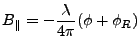(23)

So the reference phase has to be added again in order to compute Bpar. (otherwise Bpar is 0.001 m or so.)

Processing:

1. per line compute B,alpha;
2. per pixel
1. phi to Bpar
2. r known
3. compute theta (exact?)
4. compute p
5. compute mu
6. compute H
7. compute h

The idea is to compute H from the position of M. (H can be computed by (reference needed) as shown.) And then to find theta=f(B,phi,r). And then find h with the first equation. This method is implemented to check our exact method, the results are very different.

In this method the point P does not have to be computed. (though in order to compute baseline components we will compute a point P on height h (evaluate, iteratively) once for every line.)

A better way might be to use the co-registration model to compute the point S.Next: Method schwabisch Up: Implementation Previous: Method ambiguity   Contents
Leijen 2009-04-14